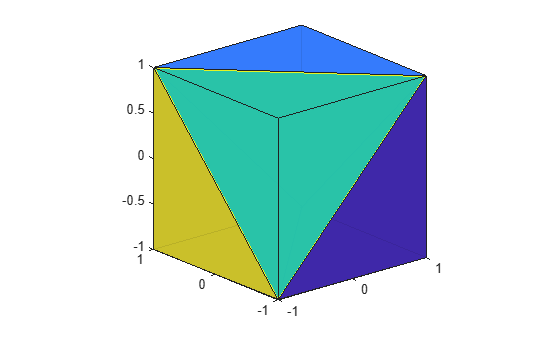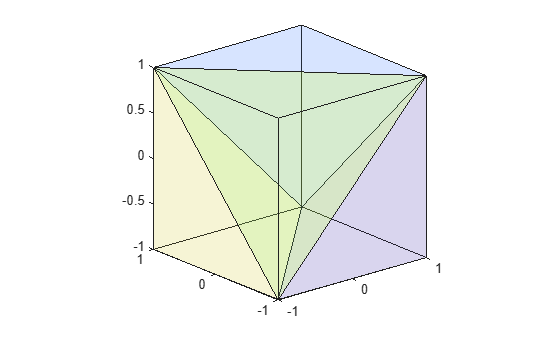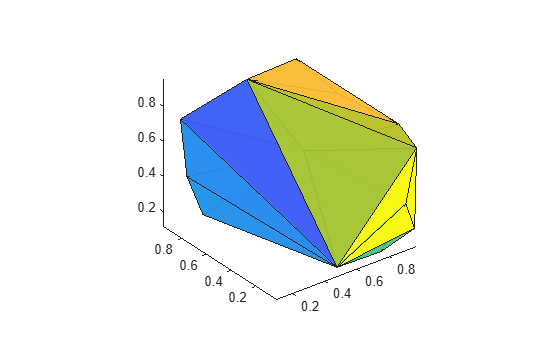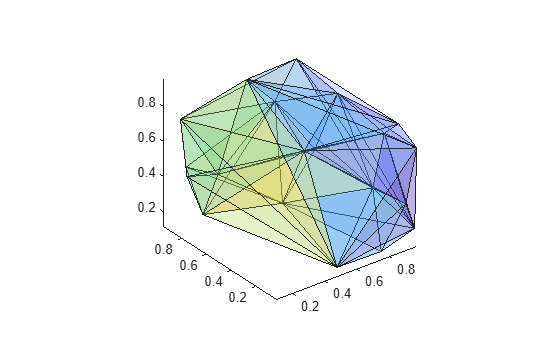tetramesh

Tetrahedron mesh plot

Description

example

tetramesh(T,X) plots the 3-D tetrahedron mesh defined by the tetrahedron connectivity matrix T and the points X.

T is an m-by-4 matrix that specifies the vertices of m tetrahedra. X is an n-by-3 matrix that specifies the Cartesian coordinates of n points. Each row of T contains indices into X that specify the 4 vertices of a tetrahedron.

example

tetramesh(TR) plots the tetrahedron mesh defined by a 3-D triangulation or delaunayTriangulation object.

example

tetramesh(___,c) also specifies the face color of each tetrahedron using a vector of colormap indices.

example

tetramesh(___,Name,Value) specifies one or more properties of the mesh plot using name-value arguments. For example, 'LineWidth',2 sets the edge width to 2 points.

h = tetramesh(___) returns a vector of tetrahedron handles. Each handle of h is a patch object used to create the mesh plot of each tetrahedron. Use h(i) to query and modify properties of the plot of each tetrahedron. For more information, see Patch Properties.

Examples

collapse all

Create 8 points that represent the corners of a cube in Cartesian coordinates.

d = [-1 1];
[x,y,z] = meshgrid(d);
X = [x(:) y(:) z(:)]
X = 8×3

-1    -1    -1
-1     1    -1
1    -1    -1
1     1    -1
-1    -1     1
-1     1     1
1    -1     1
1     1     1

Specify a tetrahedron connectivity matrix where each row contains the indices into X that specify the 4 vertices of a tetrahedron. For instance, the first row describes a tetrahedron with vertices consisting of the first, third, fourth, and seventh points.

T = [1 3 4 7;
4 6 7 8;
1 5 6 7;
1 2 4 6;
1 4 6 7]
T = 5×4

1     3     4     7
4     6     7     8
1     5     6     7
1     2     4     6
1     4     6     7

Use tetramesh to plot the 5 tetrahedra that form the cube.

tetramesh(T,X);
view(-40,20)To see into the cube, plot it again with more transparent faces.

tetramesh(T,X,'FaceAlpha',0.1);
view(-40,20)Create a 3-D delaunayTriangulation object for 20 random points.

rng('default');
x = rand([20 1]);
y = rand([20 1]);
z = rand([20 1]);
DT = delaunayTriangulation(x,y,z)
DT =
delaunayTriangulation with properties:

Points: [20x3 double]
ConnectivityList: [53x4 double]
Constraints: []

Plot the tetrahedron mesh.

tetramesh(DT)Change the color of the tetrahedra. Plot it again with the new colors and with more transparent faces.

tetramesh(DT,106:-2:1,'FaceAlpha',0.1)Input Arguments

collapse all

Tetrahedron connectivity, specified as a 4-column matrix. Each row of T contains indices into X that specify the 4 vertices of a tetrahedron.

Coordinates of points, specified as a 3-column matrix.

3-D triangulation object, specified as a triangulation or delaunayTriangulation object.

Face color, specified as a vector of colormap indices of the same size as the number of tetrahedra. The tetrahedron colors are defined by the vector c, which is used as indices into the current colormap.

To customize the color scheme, use the colormap function. For additional control over the surface coloring, use the 'EdgeColor' and 'FaceColor' name-value arguments.

Name-Value Arguments

Specify optional comma-separated pairs of Name,Value arguments. Name is the argument name and Value is the corresponding value. Name must appear inside quotes. You can specify several name and value pair arguments in any order as Name1,Value1,...,NameN,ValueN.

Example: tetramesh(TO,'LineStyle',':')

The properties listed here are only a subset. For a complete list, see Patch Properties.

Face color, specified as the comma-separated pair consisting of 'FaceColor' and a color name, an RGB triplet, or 'none'.

An RGB triplet is a three-element row vector whose elements specify the intensities of the red, green, and blue components of the color. The intensities must be in the range [0,1]; for example, [0.4 0.6 0.7]. This table lists the long and short color name options and the equivalent RGB triplet values.

Long NameShort NameRGB Triplet
'yellow''y'[1 1 0]
'magenta''m'[1 0 1]
'cyan''c'[0 1 1]
'red''r'[1 0 0]
'green''g'[0 1 0]
'blue''b'[0 0 1]
'white''w'[1 1 1]
'black''k'[0 0 0]

Face transparency, specified as the comma-separated pair consisting of 'FaceAlpha' and a scalar in the range [0,1]. A value of 1 is opaque and 0 is completely transparent. Values between 0 and 1 are semitransparent.

Edge color, specified as the comma-separated pair consisting of 'EdgeColor' and a color name, an RGB triplet, or 'none'. The default color of [0 0 0] corresponds to black boundaries.

An RGB triplet is a three-element row vector whose elements specify the intensities of the red, green, and blue components of the color. The intensities must be in the range [0,1]; for example, [0.4 0.6 0.7]. This table lists the long and short color name options and the equivalent RGB triplet values.

Long NameShort NameRGB Triplet
'yellow''y'[1 1 0]
'magenta''m'[1 0 1]
'cyan''c'[0 1 1]
'red''r'[1 0 0]
'green''g'[0 1 0]
'blue''b'[0 0 1]
'white''w'[1 1 1]
'black''k'[0 0 0]

Line width, specified as the comma-separated pair consisting of 'LineWidth' and a positive numeric value in points.

Line style, specified as one of the options listed in this table.

Line StyleDescriptionResulting Line
'-'Solid line'--'Dashed line':'Dotted line'-.'Dash-dotted line'none'No lineNo line